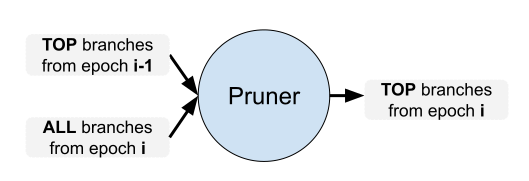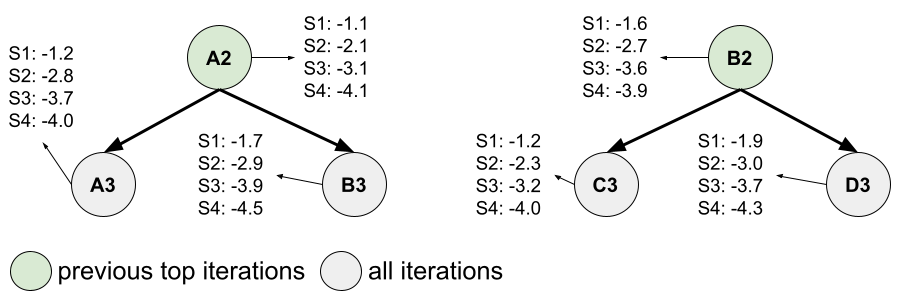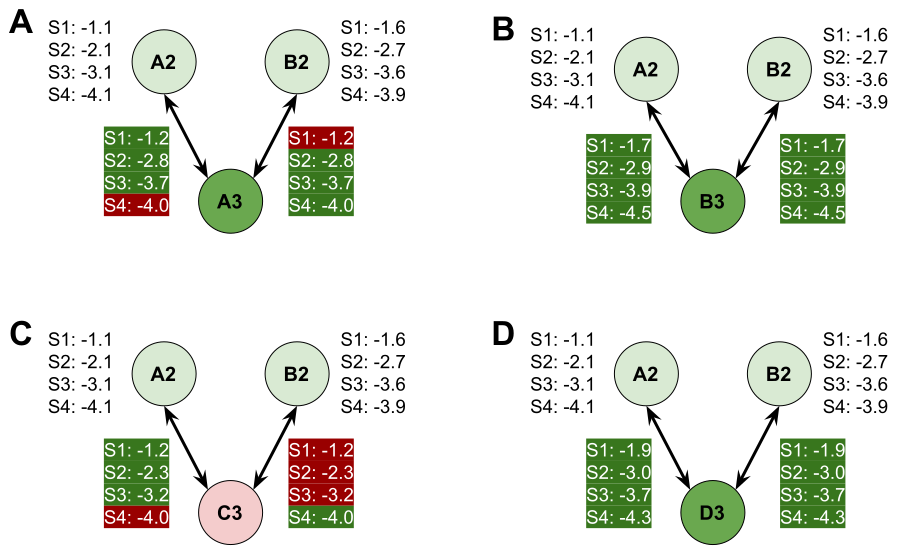# Pruners

This block is in charge of deciding which branches from the last 2 epochs go through onto the next epoch, according to their scores. It takes the top branches from the previous epoch and the current branches from the current epoch and decides which are the top branches of the current epoch.Figure 1: inputs and outputs of a pruner at epoch i. The exact process by which the pruner compares branches to decide which ones improve the affinity and should be used as a substrate for the next mutations depended on the chosen pruner.

Warning

For the purposes of these docs, we’ll be using complex and branch as synonyms, though technically, a branch is comprised of a complex and other information, like its trajectory, its scores, etc…

## locuaz.prunerconsensus module

Under this pruner, for a new complex to pass onto the next epoch, it has to beat all previous complexes that gave rise to it. Using this pruner, a new complex beats a previous one when the means of its scores are lower than those from the previous complex, given that a lower score number indicates higher affinity between the target and the binder.

Now, since scorers tend to diverge, it’s not necessary for all of them to improve. The user can set a minimum threshold of scorers that have to improve so a binder can be considered better than another.

For example, if a user is using 4 scorers, the user can set the threshold to, say, 2 and if 2 scorers indicate an improvement, then the new complex beats the old one. More formally:

$\begin{split}c_{k}^{i} = \left\{ \begin{array}{l} 1, \quad \text{if} \ avg\left(score^{i+1}_{k}\right) < avg\left(score^{i}_{k}\right) \\ 0, \quad \text{else} \end{array} \right.\end{split}$

Where $$c_{k}^{i}$$ counts how many scorers improved with respect to the previous complex, while $$k$$ and $$i$$ are the indices for scorers and complexes, respectively.

Then, a consensus number $$C^{i}$$ is obtained by adding all the $$c_{k}^{i}$$ for the $$N$$ scorers, and this number is compared against the threshold $$T$$:

$C^{i} = \sum_{k=1}^{N} c_{k}^{i}$
$C^{i} \geq T ?$

If the last statement is true, then complex $$i+1$$ beats $$i$$ and can be considered for a next round of mutations.

### consensus run example

Recapping the hypothetical example from Platform DAGs, we are going to focus on epoch 3 to see how the pruner decides that branches A3, B3 and C3 should go through, but not D3.

In this example the user selected the options pruner: consensus, consensus_threshold: 3, branches: 2 and constant_width: False, among others. Check the tutorials and the YAML configuration file if you need a refresh on these options. Figure 2 focuses on this step.Figure 2: at epoch 3, epoch 2 and 3 are considered. Since we’re going back to epoch 3, A3, B3, C3 and D3 show up in gray, as the pruner hasn’t decided yet which ones improve on the affinity and which ones don’t. Average scores according to each of the scoring functions (S1, S2, S3 and S4) show up on the side.

After the MD is ran and the scoring is done, it’s time for the pruner. The consensus pruner does the following: for each branch on the current epoch being pruned, its average scores are compared against the scores of all the top branches from the previous epoch. For example, Figure 3A shows the comparison of A3 branch against the 2 previous top branches. Since on both cases it beats the previous top branch in 3 out of 4 scorers, A3 branch will be the first top branch of epoch 3. On the other hand, we can see that C3 only improves on 1 scorer with respect to the previous top branch B2 and, hence, it can’t move forward since the user selected a consensus_threshold fo 3.Figure 3: each panel shows the comparisons between each of the epoch 3 branches and the epoch 2 top branches. Average scores highlighted in green are the ones that are better than their respective counterparts, while the red ones are worse. Branch C3 “looses” when compared against B2 and hence won’t be moving forward.

## locuaz.prunermetropolis module

When using only 1 scorer, the well known metropolis acceptance criteria can be used to decide whether a complex passes to the next epoch:

$\text{Acceptance ratio} = \min\left(1, \exp\left(-\frac{\Delta E}{k_B T}\right)\right)$

Then a random number between $$0$$ and $$1$$ is generated and if the Acceptance ratio is above it, then the new complex is considered to beat the old one.